# 一个简单算法可以帮助物联网,金融 用户 节约98%的数据存储成本 (PostgreSQL,Greenplum帮你做到)

PostgreSQL简直是工业界和学术界的完美结合，怎么说呢？

https://yq.aliyun.com/articles/18034
https://yq.aliyun.com/edu/lesson/67

5个字段，随机生成一些数据，其中一个字符串，3个INT，1个时间类型。

digoal=# create table heap_test(c1 int, c2 text, c3 int, c4 int, c5 timestamp);
digoal=#
insert into heap_test
select
random()*5000000,
repeat(md5(c::text),32),
c+random()*50000000,
random()*1000000,
current_date+(150000*random())::int
from
(select trunc(random()*100000) c from generate_series(1,50000000) t(id)) t;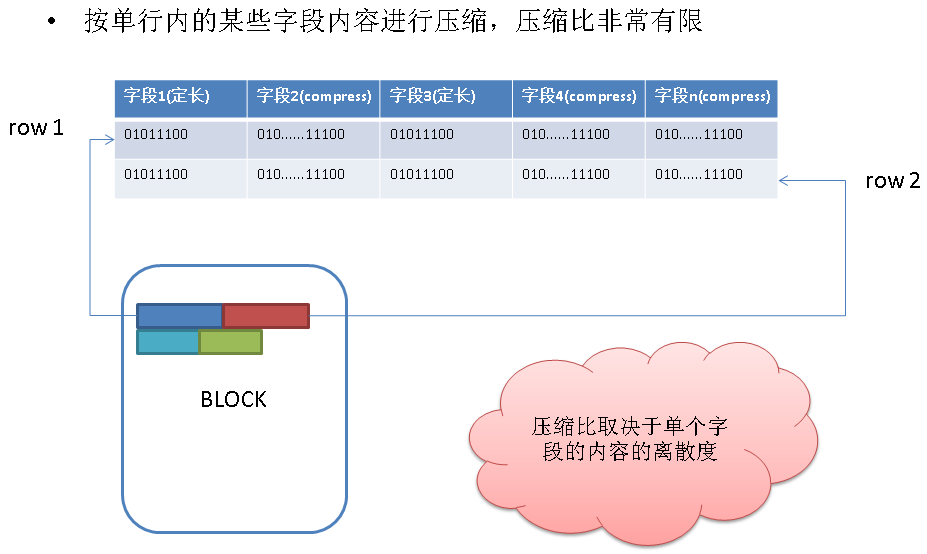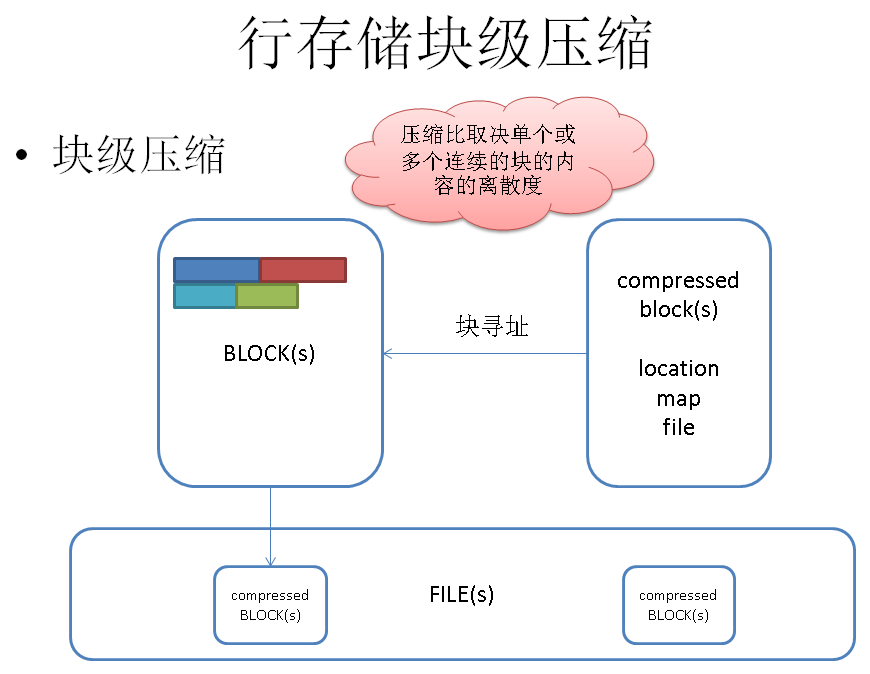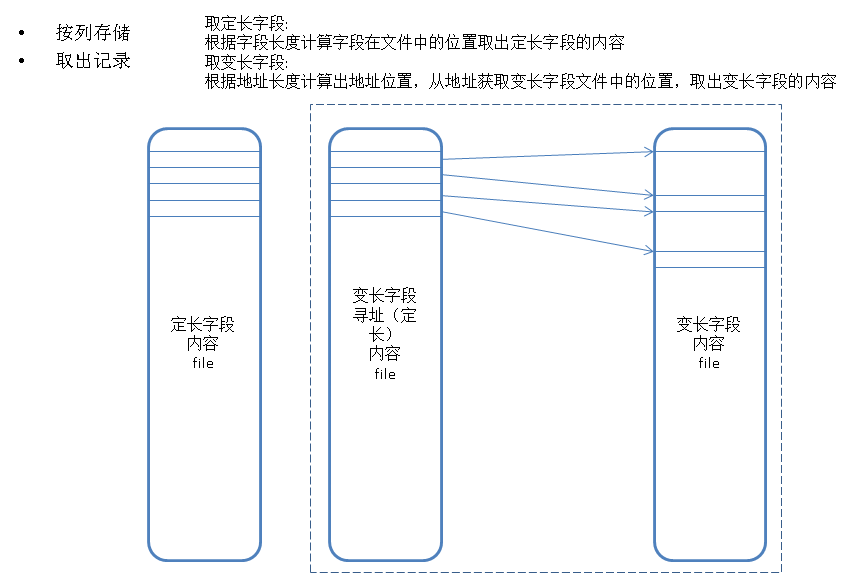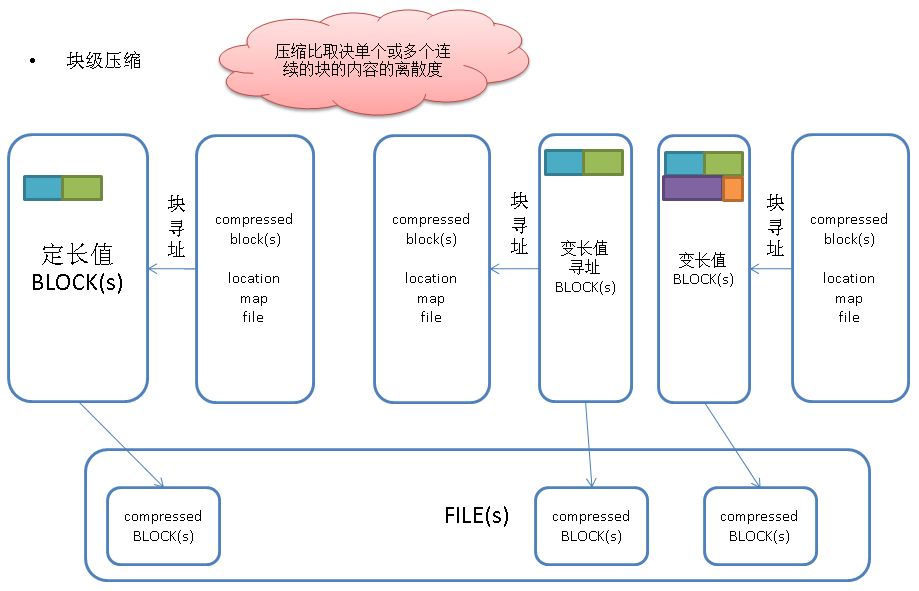digoal=# create table ao1_test(c1 int, c2 text, c3 int, c4 int, c5 timestamp) with (APPENDONLY=true,BLOCKSIZE=2097152,ORIENTATION=column,COMPRESSTYPE=zlib,CHECKSUM=false);
digoal=# insert into ao1_test select * from heap_test ;
digoal=# analyze ao1_test;
ANALYZE
digoal=# select pg_size_pretty(pg_total_relation_size('ao1_test'));
pg_size_pretty
----------------
21GB
(1 row)

.1. 字段值的冗余度，冗余度越高的值，排序后的压缩比越高。
.2. 字段值的平均宽度，平均宽度大的值，压缩取得的绝对效果比宽度小的值好。
.3. 字段与字段间的线性相关性，线性关系好的字段，按其中一个A字段排序后线性关系也好，从而相关字段B的压缩效果也会比较好，从而达到双赢的目的。

case
when n_distinct < 0 then 1 + n_distinct
when n_distinct = 1 then 1
when n_distinct > 1 then 1 - n_distinct/reltuples
end

avg(pg_column_size(column_name)

https://yq.aliyun.com/articles/18038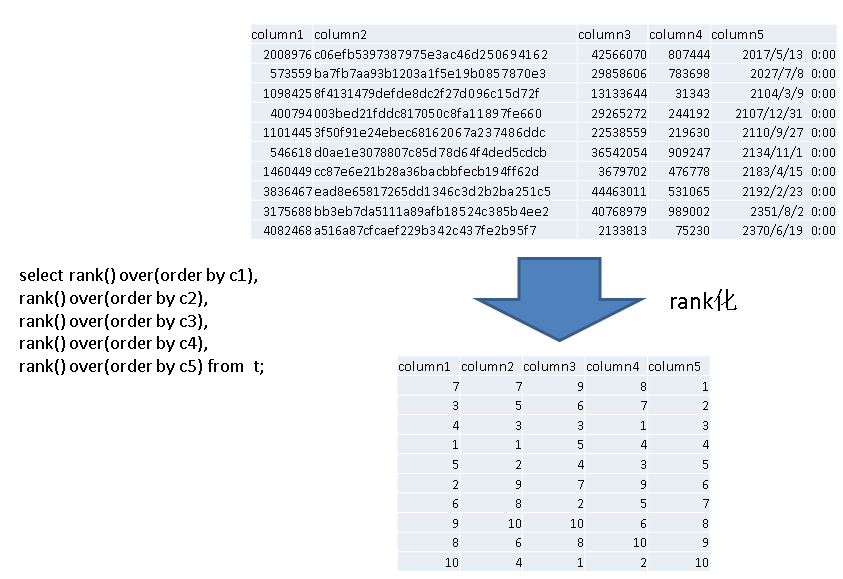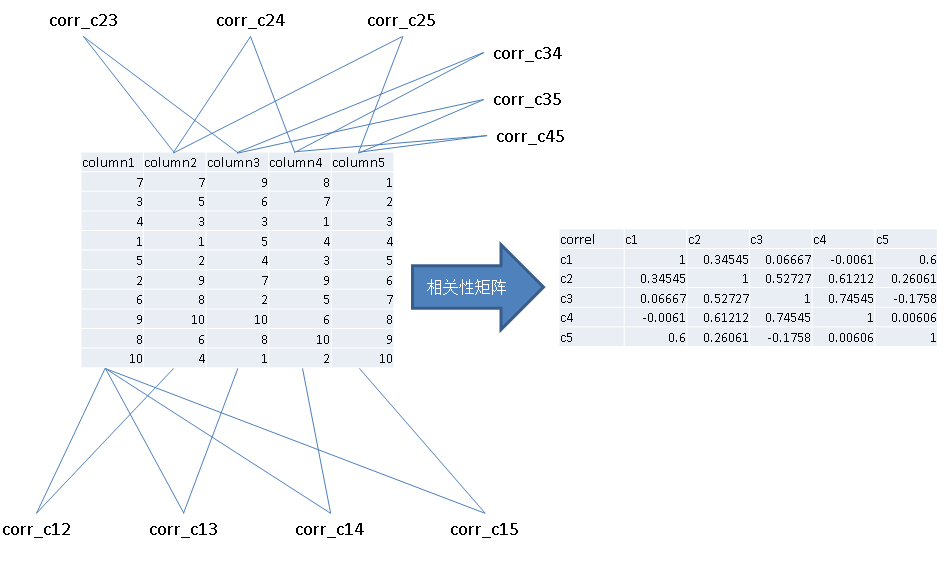https://yq.aliyun.com/articles/17228

CREATE or replace FUNCTION has_dupli_val(VARIADIC arr int[]) RETURNS boolean AS
$$select count(distinct val) <> count(*) dist_val from unnest(1) t(val) where val is not null;$$
language sql strict;

create or replace function best_compress_cols(samp int8) returns void as
$$declare cols text[] := array['c1', 'c2', 'c3', 'c4', 'c5']; -- 参与计算的字段(最多5列, 至少有一列的冗余度>10%) sub int[]; -- 计算过程中用到的数组下标 res_sub int[]; -- 计算结果用到的数组下标 comp_rat numeric; -- 计算过程中存储的压缩率 res_comp_rat numeric := 0; -- 计算结果的压缩率 cols_w_avg int[] := array[4, 1028, 4, 4, 8]; -- 参与计算的字段, 对应的平均宽度 dup_rat numeric[] := array[0.941288981586695, 0.999979166666666666666667, 0.996877203229815, 0.999979166814228, 0.999697591731092]; -- 参与计算的字段, 对应的冗余度 corr numeric[][]; begin -- 计算字段之间的相关性, 存入矩阵corr[][] execute ' select array[ array[1,c12,c13,c14,c15] , array[c12,1,c23,c24,c25] , array[c13,c23,1,c34,c35] , array[c14,c24,c34,1,c45] , array[c15,c25,c35,c45,1] ] from ( select corr(c1,c2) c12, corr(c1,c3) c13, corr(c1,c4) c14, corr(c1,c5) c15, corr(c2,c3) c23, corr(c2,c4) c24, corr(c2,c5) c25, corr(c3,c4) c34, corr(c3,c5) c35, corr(c4,c5) c45 from ( select rank() over(order by c1) c1, rank() over(order by c2) c2, rank() over(order by c3) c3, rank() over(order by c4) c4, rank() over(order by c5) c5 from ( select ' || cols||' c1, '||cols||' c2, '||cols||' c3, '||cols||' c4, '||cols||' c5 ' || ' from heap_test order by random() limit ' || samp || ' ) t ) t ) t ' into corr; -- 生成字段序号排列组合 for sub in with t(id) as (select generate_series(1,5)) select array[t1.id,t2.id,t3.id,t4.id,t5.id] from t t1,t t2,t t3,t t4,t t5 where not has_dupli_val(t1.id,t2.id,t3.id,t4.id,t5.id) LOOP comp_rat := abs( cols_w_avg[sub] * dup_rat[sub] * corr[sub][sub] )+ abs( cols_w_avg[sub] * dup_rat[sub] * corr[sub][sub] )+ abs( cols_w_avg[sub] * dup_rat[sub] * corr[sub][sub] )+ abs( cols_w_avg[sub] * dup_rat[sub] * corr[sub][sub] )+ abs( cols_w_avg[sub] * dup_rat[sub] * corr[sub][sub] ); raise notice '%, %, %, %, %, compress ratio: % ', cols[sub], cols[sub], cols[sub], cols[sub], cols[sub], comp_rat; if res_comp_rat = 0 then res_comp_rat := comp_rat; res_sub := sub; elseif comp_rat > res_comp_rat then res_comp_rat := comp_rat; res_sub := sub; end if; END LOOP; -- 输出压缩比最大的组合 raise notice 'resutl: %, %, %, %, %. compress ratio: % ', cols[res_sub], cols[res_sub], cols[res_sub], cols[res_sub], cols[res_sub], res_comp_rat; end;$$
language plpgsql strict;

select best_compress_cols(50000000);  --  采样5000万记录，计算最佳压缩排序.

NOTICE:  resutl: c2, c4, c3, c5, c1. compress ratio: 1031.11112165362824768278103927944140

digoal=# create table ao2_test(c1 int, c2 text, c3 int, c4 int, c5 timestamp) with (APPENDONLY=true,BLOCKSIZE=2097152,ORIENTATION=column,COMPRESSTYPE=zlib,CHECKSUM=false);
digoal=# insert into ao2_test select * from heap_test order by c2, c4, c3, c5, c1 ;
digoal=# analyze ao2_test;
ANALYZE
digoal=# select pg_size_pretty(pg_total_relation_size('ao2_test'));
pg_size_pretty
----------------
4603MB
(1 row)

https://yq.aliyun.com/articles/18034
https://yq.aliyun.com/edu/lesson/67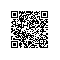使用钉钉扫一扫加入圈子
+ 订阅